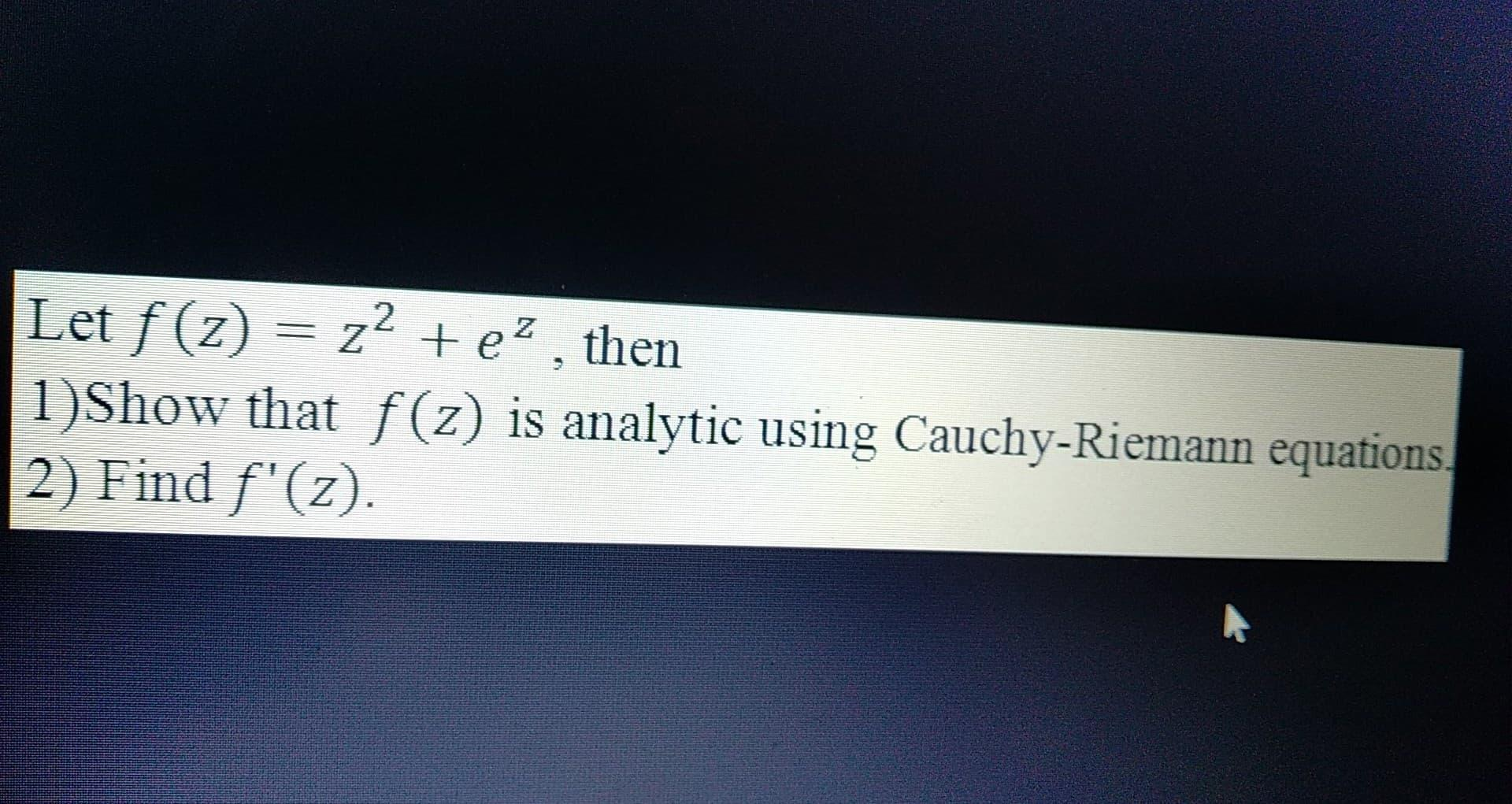### Create an Account

Already have account?

### Forgot Your Password ?

Home / Questions / Let f (z) = z2 +e?, then 1)Show that f(z) is analytic using Cauchy-Riemann equations 2) Find f'(z)

# Let f (z) = z2 +e?, then 1)Show that f(z) is analytic using Cauchy-Riemann equations 2) Find f'(z)

Let f (z) = z2 +e?, then 1)Show that f(z) is analytic using Cauchy-Riemann equations 2) Find f'(z)Apr 14 2021 View more View Less

#### Answer (Solved)Subscribe To Get Solution